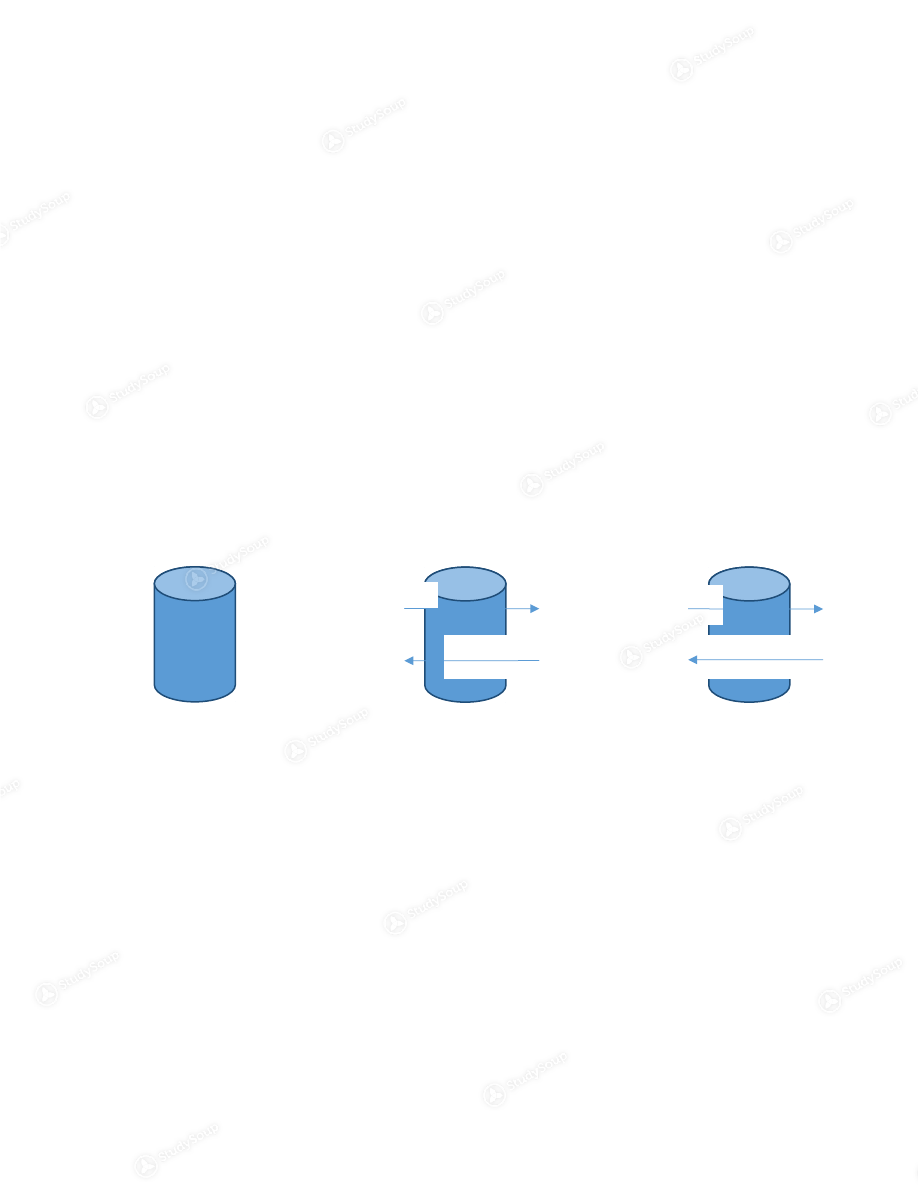×

# UA - CH 102 - CH 102 | Final Study Guide - Study Guide

### Created by: Isabella Ryerson Elite Notetaker

> > > > UA - CH 102 - CH 102 | Final Study Guide - Study Guide

UA - CH 102 - CH 102 | Final Study Guide - Study Guide

##### Description: This is the study guide for the final on December 7, 2016.
0 5 3 23 Reviews
This preview shows pages 1 - 6 of a 33 page document. to view the rest of the contentFinal Exam Study Guide CH 102 Lectures 1, 2, & 3: Phases of Matter and Intermolecular Forces Describe the differences between the phases of matter (solids, liquids,
and gasses).
Solids: high density, fixed shape, fixed volume, intermolecular  forces strong. A solid is a solid because the molecules are being held
together and the thermal motion/energy of the molecules
cannot overcome intermolecular forces
Gasses: low density, shape not fixed, volume not fixed,  intermolecular forces weak.  A gas is a gas because the molecules are not being held
together and thermal motion/energy of the molecules does
overcome the intermolecular forces
Liquids: density moderately high, shape not fixed, fixed volume, intermolecular forces moderately strong. A liquid’s intermolecular strength is in between that of a
gas and a solid
Changing between states What causes intermolecular attraction? o All attractions between molecules are due to Coulombic  attractions o Caulombic attractions are just charges
o If particles are close, then the attraction is strong
o If particles are far away, then the attraction is weak
o Larger opposite charges = more attraction
List the types of intermolecular forces in order of weak to strong and
describe them at the molecular level
Dispersion Forces (London Forces):  Attractive forces that arise as a result of temporary dipoles
induced in atoms or molecules
all molecules are subject to dispersion forces the instantaneous dipole of one molecule can induce a
dipole in neighboring atoms (look at my “Lecture 1” notes
to see an illustration of this)
heat or reduce
pressure
heat GA
S
LIQUI
D
SOLI
D
cool cool or increase
pressureit is the attraction of these dipoles that cause dispersion
forces
as molar mass increases, so does the boiling point  this is because the magnitude of the dispersion force will
increase with more electrons that can move around easily
larger electron cloud = larger dispersion forces generally, higher molecular weights = larger dispersion
forces
Polarizability: how easy the electron distribution in the
atom or molecule can be distorted (or “pushed around”)
Increases with: greater number of electrons, more
diffuse the electron could
Dipole-Dipole Forces: Attractive forces between polar molecules Many molecules have permanent dipoles Molecules with permanent dipoles have a stronger
attraction to each other
Hydrogen Bonding: A special dipole-dipole interaction between the hydrogen
atom in a polar N-H, O-H, or F-H bond and an
electronegative O ,N, or F atom
Ion-Dipole Forces: Attractive forces between an ion and a polar molecule o Ion-Ion Forces: Attractive forces between ions Lecture 3: Surface Tension, Viscosity, and Capillary Action Define and explain molecular factors that influence tension, viscosity,
and capillary action
Surface Tension the tendency of liquids to minimize surface area measured in mJ/ m^2 how much surface area is takes to increase the surface
area of a liquid
think about how you see bugs running on top of a pond generally, the higher the surface tension, the
stronger the intermolecular forces
Viscosity the resistance of a liquid to a flow measured in units of centipoise (cP)=g*cm^-1*s^-1) think of the thickness of honey and how it takes a long
time to pour
Decreases with increasing temperature Capillary actioncombination of intermolecular attraction of molecules in a
liquid and the attraction of a liquid to a surface
think of what happens when you touch a paper towel to
water
this also causes water to travel up narrow tubes the narrower the tube, the farther it travels Lecture 4: Vapor Pressure, Boiling Point, Critical Point, Sublimation,  Fusion, and the Heating Curve of Water Describe the process of vaporization and condensation at the
molecular level
Vaporization: molecules leave the liquid state and become a  gas Condensation: molecules leave the gas state and become a  liquid Vaporization is an endothermic process o What does endothermic mean? A system that absorbs energy from the surroundings Condensation is an exothermic process o What does exothermic mean? A system that releases energy into the surroundings  Water is held together by hydrogen bonds o A certain amount of energy is required to overcome the  hydrogen bonds o At any temperature, some molecules have more kinetic energy  than others o Those with enough energy can escape and form a vapor Heat of Vaporization (enthalpy of vaporization) o Amount of energy needed to vaporize 1 mol of a liquid to a gas
Always exothermic
o Know how to calculate this
This is a dimensional analysis problem You will be given the  H vap  Your answer will be in kJ You can see example problems in my “Lecture 4” notes Apply the concept of dynamic equilibrium to the vapor pressure in a
closed system
Dynamic Equilibrium: the rate of evaporation is equal to the  rate of condensation o So in a closed system in dynamic equilibrium, the number of  water molecules that are vaporizing is equal to the number of
molecules condensing
o Think about pulling a piston up: volume increases, pressure falls,  more gas vaporizes, and then the pressure is restoredo Then think about pushing a piston down: volume is decreased,  pressure rises, more gas condenses, and then pressure is
restored
o There is an illustration in this in our powerpoint and in my  “Lecture 4” notes o This piston example perfectly explains Le Chatelier’s  Principle: When a system in a dynamic equilibrium is disturbed, the
system responds so as to minimize the disturbance and
o Temperature Dependence As you increase temperature, the vapor pressure increases
until it reaches its boiling point.
Use the Clausius-Clapeyron equation (both forms) o The Clausius-Claperion Equation relates vapor pressure to  vaporization o This is in the form of y=mx+b
o You can plot and solve for this
o 𝑙𝑙𝑙
𝑙𝑙𝑙  = (−∆𝑙 𝑙𝑙𝑙/  𝑙𝑙) + ln 𝑙 o 𝑙𝑙𝑙 𝑙𝑙𝑙  = (−∆𝑙 𝑙𝑙𝑙/  𝑙) (1/ 𝑙) + ln 𝑙 o You can see a worked out example in my “Lecture 4” notes Describe the critical point o A point on a phase diagram at which both the liquid and gas
phases of a substance have the same density, and are therefore
indistinguishable
What is sublimation? o Direct transition of a solid to a gas o Think of dry ice What is fusion? o Fancy word for melting o Heat of fusion (∆𝑙 fus ) The energy required to melt one molecule of a substance The Heating Curve of Water o You can see a good diagram of this in my “Lecture 4” notes
o Or you can google it and look at those diagrams (those may
exclude some of the info Dr. Rupar provided in class and expects
us to know)
Lecture 5: Phase Diagrams Calculate the quantify the heat needed for a substance to undergo
phase transitions
o You can see a worked out example in my “Lecture 5” notes What is a phase diagram?o a diagram representing the limits of stability of the various
phases in a chemical system at equilibrium, with respect to
variables such as composition and temperature
o You can see a good diagram of this in my Lecture 5 notes taken  on September 1, 2016 o Or you can google it and look at those diagrams. Just make sure  the picture you study includes all of the “key parts” listed below Describe key parts of a phase diagram y-axis: pressure
x-axis: temperature
Gas: low pressure, high temperature
Liquid: increase pressure
Solid: increase pressure, lower temperature
Critical Point: the point where the distinction between liquids
and gasses cease to exist Fusion Curve: liquid/solid barrier, also known as  freezing/melting Vaporization Curve: liquid/vapor barrier, also known as  evaporation/condensation Sublimation Curve: solid/vapor barrier
Triple Point: the temperature and pressure at which the three
phases (gas, liquid, and solid) of that substance coexist in
thermodynamic equilibrium
Use a phase diagram to predict phase changes in a substance What makes the phase diagram of water special? o Water expands when it freezes What is regelation? o Melting ice by pressure Lecture 6: Crystalline Solids Qualitatively describe x-ray crystallography  o X-ray particles go under constructive (dot) and destructive (not  dot) interference o Constructive interference occurs when Bragg’s Law is satisfied What is Bragg’s Law? o there is a definite relationship between the angle at which a
beam of x-rays must fall on the parallel planes of atoms in a
crystal in order that there be strong reflection
Use Bragg’s law to calculate spacing at the atomic level  o n =2dsin o Look in my “Lecture 6” notes for a worked out example What is a crystal lattice? o the symmetrical three-dimensional arrangement of atoms inside
a crystalo a crystal lattice is made from unit cells   What is a unit cell? o A particular arrangement of atoms that repeat over and over and over again to form a crystal lattice o Tiles on a bathroom floor are the unit cells in a 2D space Describe the three cubic unit cells (way more notes on this in “Lecture
6” notes and in “Recitation 2” notes)
Simple Cubic Unit Cell: atoms at each corner coordination number of 6, packing efficiency of 52%, and
contains 1 atom per unit cell
o Body-Centered Unit Cell: Atoms at each corner and one in the center Coordination number of 8, packing efficiency of 68%, and
contains 2 atoms per unit cell
Face-Centered Unit Cell: Atoms at each corner and one on each face Coordination number of 12, packing efficiency of 74%, and
contains 4 atoms per unit cell
What is a coordination number? o The number of atoms with which each atom is in contact with What is packing efficiency? o The % of volume of the unit cell occupied by the atoms
o The higher the coordination number, the higher the packing
efficiency Lecture 7: Classifying Crystalline Solids | Solutions and Solubility Describe the three crystalline solids Molecular Solids: Made from molecules Geld together by dipole-dipole bonds, hydrogen bonds, and
dispersion forces
They have a low melting point CO 2  and H 2 O are good examples o Ionic Solids: Made up of cations and anions They have a high melting point Interactions are much stronger NaCl is a good example Atomic Solids: Non-Bonding Atomic Solids: Held together by dispersion forces Very low melting points

This is the end of the preview. Please to view the rest of the contentJoin more than 18,000+ college students at University of Alabama - Tuscaloosa who use StudySoup to get ahead
33 Pages 119 Views 95 Unlocks
• Notes, Study Guides, Flashcards + More!Join more than 18,000+ college students at University of Alabama - Tuscaloosa who use StudySoup to get ahead